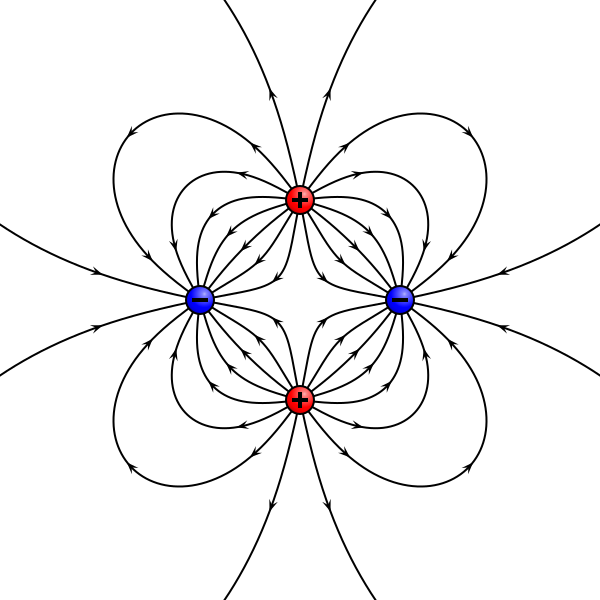# Electric Field LinesElectric Field Lines are imaginary curves drawn in space surrounding a given charge distribution. Observing these electric field lines, the nature of electric field i.e. its direction and relative strength can be estimated in a very simple manner. The electric field lines are hypothetical curves providing an overall pictorial representation of the electrostatic field.

It was Michael Faraday, in 19th century who first proposed the concept of electric field lines. He always thought of the electric field lines as ones that can be used to describe and interpret the invisible electrostatic field. It is always convenient to use electric field lines to analyze the field around a system of charges instead of using complex vector diagram each time.

### Properties of Electric Field Lines

Some general properties and facts regarding electric field lines are mentioned below:

1. The tangent to an electric field line at any point gives the direction of resultant electric field vector at that point. This is also the path on which a positive test charge will tend to move if free to do so.
2. The relative density of electric field lines near any two points gives an idea about the magnitudes of electric fields at those points. In other words, a denser field lines represent a higher field strength.
3. Two electric field lines can never intersect each other as there cannot be two directions of resultant electric field at one point.
4. Electric field lines always flow from higher potential to lower potential.
5. Electric field lines are absent in a zero electric field region.
6. The electric field lines are continuous and smooth in a charge-free region.
7. Electric field lines always emerge from a positive charge and terminate on a negative charge. No field line can originate or terminate in the space surrounding a charge. In case of a single positive charge field lines end at infinity.
8. The number of electric field lines originating from a positive charge or ending at a negative charge is directly proportional to the magnitude of the charge.
9. One is free to draw any number of electric field lines around a charge but the ratio of magnitude of charge to the number of field lines is to be kept fixed. For example, if 100 electric field lines are emerging from +1C charge, then 100 will terminate on -1C charge. Similarly if one chooses to draw 40 field lines beginning from +4μC charge he will have to consider 20 field lines terminating on -2μC charge or 10 field lines terminating on -1C charge and so on.
10. An electric field line can never form a closed loop as it cannot start and end on same charge. This is also because the electric field is conservative in nature.
11. The number of electric field lines passing through a surface is the measure of the of the electric flux linked with the surface. If same number of field lines cross through two different surfaces, flux through both the surfaces would be equal.
12. If there is an equipotential surface, the electric field vector and hence lines of electric field will be perpendicular to the surface.
13. In a uniform electric field region, the electric field lines are parallel and equi-spaced straight lines.

### Nature of Electric Field Lines for uniform and non-uniform Fields

The electric field in a space around a charge distribution can be of two types. These are the uniform electric field and the non-uniform field.

### Uniform Electric Field

When in a particular region of space, the magnitude and direction of the electric field intensity vector is same at each and every point, then the electric field in that region is said to be of uniform nature. In such region, the electric field vector does not vary with position.
As stated earlier, the electric field lines for uniform electric field are parallel and equidistant straight lines. The parallel lines indicate the unique direction of the field while their equi-spacing ensures the uniform magnitude of the field strength.

Such a uniform electric field can exist in the space between the plates of a parallel plate capacitor or near a large thin sheet of uniform areal charge density.

#### Non-uniform Electric Field

When the electric field is irregular at every point, it is called non-uniform electric field. In most of the cases, the electric fields are generally non-uniform in nature. Some examples of non-uniform field are : field due to a point charge, field produced by an electric dipole, field due to a charged ring etc. The field lines may be in the form of straight lines or irregular (but Continuous) curves representing the varying nature of field strength. The non-uniform electric field produced by a typical system of four point charges is shown below.Hindi Hearts